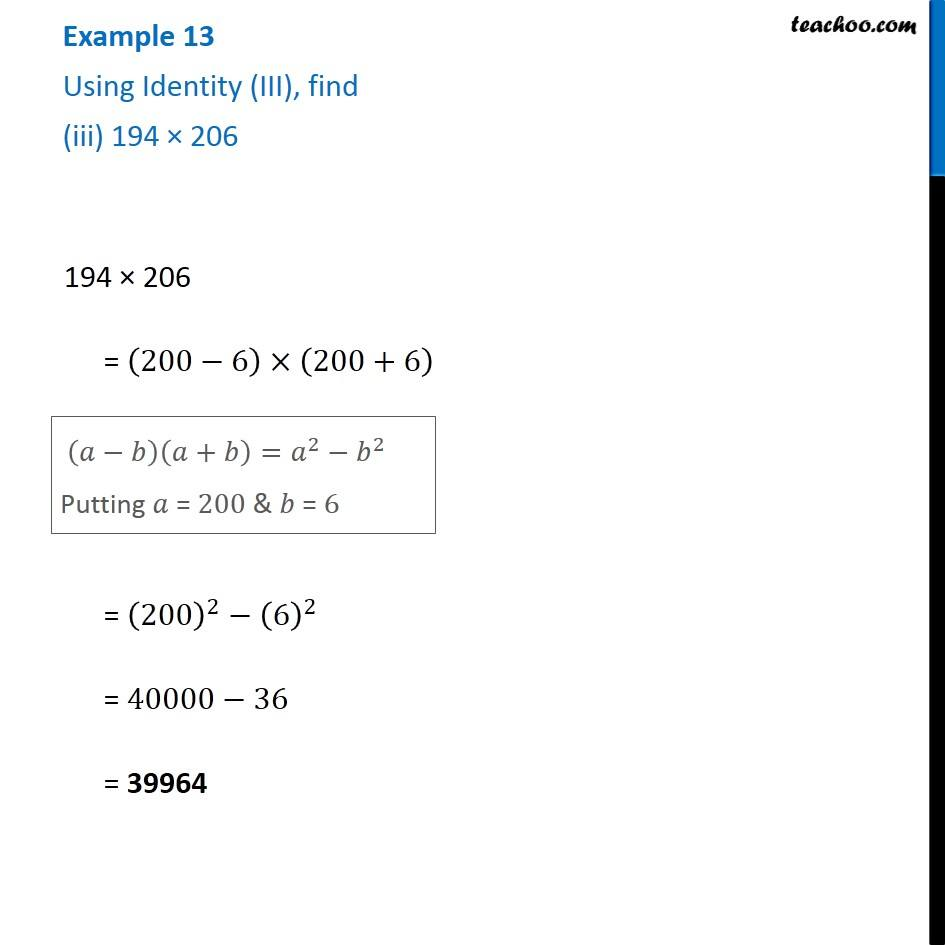Examples

Chapter 8 Class 8 Algebraic Expressions and Identities
Serial order wiseLearn in your speed, with individual attention - Teachoo Maths 1-on-1 Class

### Transcript

Example 13 Using Identity (III), find (iii) 194 × 206 194 × 206 = (200−6)×(200+6) 194 × 206 = (200−6)×(200+6) = (200)^2−(6)^2 = 40000−36 = 39964